﻿ 强阻尼波动方程的近似惯性流形 Approximate Inertial Manifold of Strongly Damped Wave Equation

Pure Mathematics
Vol.05 No.06(2015), Article ID:16436,6 pages
10.12677/PM.2015.56040

Approximate Inertial Manifold of Strongly Damped Wave Equation

Sufang Zhang, Jianwen Zhang

College of Mathematics, Taiyuan University of Technology, Taiyuan Shanxi

Received: Nov. 4th, 2015; accepted: Nov. 21st, 2015; published: Nov. 27th, 2015ABSTRACT

In this paper, the global attractor approximation by smooth manifold is considered in strongly damped equation. A nonlinear approximate inertial manifold of strongly damped wave equation is constructed. The order of approximation of the inertial manifold to the global attractor is obtained．

Keywords:Strongly Damped, Approximate Inertial Manifold, Wave Equation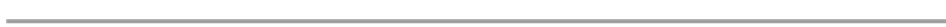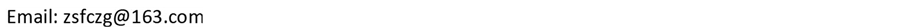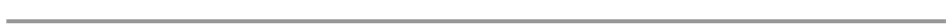1. 引言(1)

2. 预备知识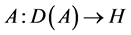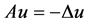，则为无界正自共轭算子，原方程(1)可化为如下的微分系统：(2)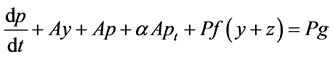(3)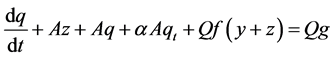(4)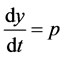(5)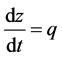(6)(7)(8)(9)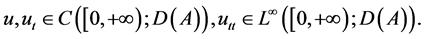3. 主要结果及其证明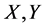为banbch空间，空间模分别记为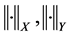，对任意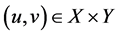，空间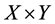上的模记为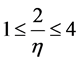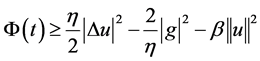(10)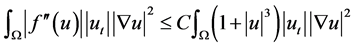(11)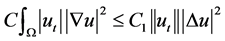(12)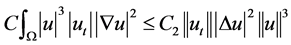(13)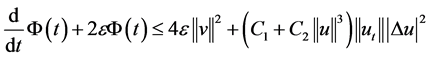(14)(15)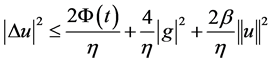(16)，此时(14)式可以重写为(17)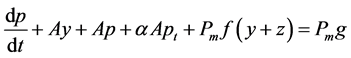(18)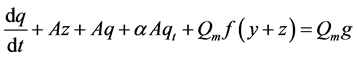(19)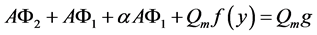(20)(21)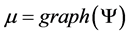是原方程的近似惯性流形。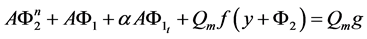(22)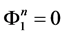(23)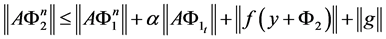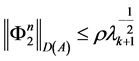(24)(25)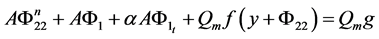(26)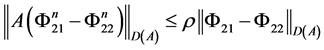(27)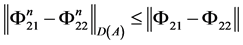(28)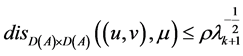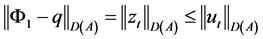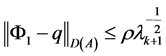(29)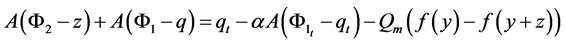(30)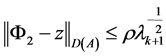(31)

Approximate Inertial Manifold of Strongly Damped Wave Equation[J]. 理论数学, 2015, 05(06): 278-283. http://dx.doi.org/10.12677/PM.2015.56040

1. 1. Clarkson, P.A., Leveque, R.J. and Saxton, R.A. (1986) Solitary-Wave Interaction in Elastic Rods. Studies in Applied Mathematics, 75, 95-122. http://dx.doi.org/10.1002/sapm198675295

2. 2. Seyler, C.E. and Fanstermacher, D.L. (1984) Asymmetric Regularized Long Wave Equation. Physics of Fluids, 27, 58-66.

3. 3. Xie, Y.Q. and Zhong, C.K. (2009) Asymptotic Behavior of a Class Nonlinear Evolution Equation. Nonlinear Analysis, 71, 5095-5105. http://dx.doi.org/10.1016/j.na.2009.03.086

4. 4. Xie, Y.Q., Yang, L. and Qin, G.X. (2007) Strain Solitary Waves in a Nonlinear Elastic Rod. Journal of Hunan University, 34, 58-61.

5. 5. Foias, C., Sell, G. and Temam, R. (1985) Varies Inertilles des Equations Differentials Dissipative. C. R. Acad Sci. Paris Ser I Math, I301, 139-142.

6. 6. 蒲志林, 陈光淦. 非线性Schodinger-Boussnesq的近似惯性流形[J]. 四川师范大学学报: 自然科学版, 2005, 28(4): 419-421.

7. 7. 魏赟赟, 陈光淦. 非自治反应扩散方程的近似惯性流形[J]. 四川师范大学学报: 自然科学版, 2005, 28(4): 419-421.

8. 8. Xie, Y.Q. and Zhong, C.K. (2007) The Existence of Global Attractors for a Class Nonlinear Evolution Equation. Journal of Mathematical Analysis and Applications, 336, 54-69. http://dx.doi.org/10.1016/j.jmaa.2006.03.086